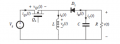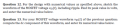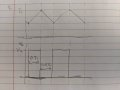# Buck Boost Converter MOSFET Voltage

#### andrep182

Joined Feb 7, 2012
6
Hi all,

First time posting in this thread, hoping I can get help on a homework I'm stuck with.Working on a homework on buck-boost, given the following :
• Vin = 12V
• Vout = -5V
• R load = 2.5 ohm
• Fsw = 20kHz
• Assume ideal, at CCM
I have calculated more values based on some of the questions it asked:
1. Duty cycle = 0.2941
2. Inductor current = 2.833A
3. Inductor value (assuming delta IL = 15% of IL) = 20.76uH
4. Capacitor value (assuming delta Vc = 25mV) = 58.82uF
I am having trouble answering the last 2 questions of this HW:My understanding is that the Vq is the switching voltage. Which means that the waveform should look something like this:Assuming that, for question 12, the peak voltage should be equal to Vin = 12V. Furthermore, for #13, my guess is the DC component of this waveform should be Vin * D = 2.99V. Of course I got both questions wrong and thus I'm here looking for advice. Not sure how else to go about this logic at the moment. Any help is appreciated, thanks!

•aashree

#### crutschow

Joined Mar 14, 2008
30,116
For starters, if you are looking for the peak voltage across the MOSFET, than it has to be more than 12V, since it also sees the negative voltage the inductor has when the MOSFET is off (to generate the -5V output), in addition to the +12V input voltage.

•savdowning

#### crutschow

Joined Mar 14, 2008
30,116
Oh right! as soon as the FET turns on again, the potential on that inductor node is still -5V. So it should look like this then?

View attachment 182603

Which means the peak voltage should actually be 17V?
Yes at the instant it turns off (neglecting any diode voltage drop which would make it a little higher).

•andrep182

#### andrep182

Joined Feb 7, 2012
6
Thanks! Now, comes the next question (about the dc component part). My thought process was that it should be the average voltage of Vq, but I'm not sure how to calculate that. Any hint you can give me?

#### crutschow

Joined Mar 14, 2008
30,116
Actually the voltage when the MOSFET is off is basically a constant, since although the inductor current is changing, the voltage does not as long as it is pulling current through the diode and charging the -5V output capacitor.

Then to get the average voltage you just take the off-voltage and the on-voltage and use the duty-cycle to calculate the average.

•andrep182

#### andrep182

Joined Feb 7, 2012
6

#### MrAl

Joined Jun 17, 2014
9,166
Hi,

How did you calculate the inductor value?
I ask because 21uH sounds small for a switch frequency of 20kHz.

•Kjeldgaard

#### andrep182

Joined Feb 7, 2012
6
Hi,

How did you calculate the inductor value?
I ask because 21uH sounds small for a switch frequency of 20kHz.
Good catch, and it was a typo from my part, sorry ... Fsw is supposed to be 200kHz. That should make sense now, but in case you're still wondering what I used to calculate it, it was with the following:

L = (Vin*D*T)/(2*delta IL)

#### MrAl

Joined Jun 17, 2014
9,166
Good catch, and it was a typo from my part, sorry ... Fsw is supposed to be 200kHz. That should make sense now, but in case you're still wondering what I used to calculate it, it was with the following:

L = (Vin*D*T)/(2*delta IL)
I was going to guess either 200kHz or 210uH•andrep182

#### Uilnaydar

Joined Jan 30, 2008
118
This thread brought tears to my eyes. TS posted question, full details, and his attempt to work it out. He then engaged all the other folks in the dialog and questions. <sniff> This was BEAUTIFUL!! I would consider it an honor to interview you in the future TS!

•BobaMosfet and andrep182

#### amol2001

Joined Dec 22, 2019
1
So what is the answer of DC component part ? i tried the (Von*Don)+(Voff*Doff) minus the ripple pf 0.025mV

#### MrAl

Joined Jun 17, 2014
9,166
So what is the answer of DC component part ? i tried the (Von*Don)+(Voff*Doff) minus the ripple pf 0.025mV
Hi,

Do you know what the waveform looks like at the top of the inductor?
For example if it is rectangular when it has a min and max, and since you know the duty cycle and the other side of the switch is at a constant voltage, you should be able to calculate the average DC voltage across the switch.
Does this makes sense to you?

#### MrAl

Joined Jun 17, 2014
9,166
So what is the answer of DC component part ? i tried the (Von*Don)+(Voff*Doff) minus the ripple pf 0.025mV
Have you figured this out yet?

Think about what the voltage across the inductor has to be when the switch is on and with the switch is off when the converter operates in CCM.

#### dinuka1997

Joined Sep 15, 2020
4
Hi,

Do you know what the waveform looks like at the top of the inductor?
For example if it is rectangular when it has a min and max, and since you know the duty cycle and the other side of the switch is at a constant voltage, you should be able to calculate the average DC voltage across the switch.
Does this makes sense to you?
Please explain this. I cant understand how to get dc component.

#### MrAl

Joined Jun 17, 2014
9,166
Please explain this. I cant understand how to get dc component.
Hi,

Is this homework for you also?

#### dinuka1997

Joined Sep 15, 2020
4
Hi,

Is this homework for you also?
Hi,
Yes. Im new to this part. And I cant get the answer. Can anyone help me to understand this?
How the other side voltage affect this?
thanks...

#### dinuka1997

Joined Sep 15, 2020
4
Hi,
Yes. Im new to this part. And I cant get the answer. Can anyone help me to understand this?
How the other side voltage affect this?
thanks...
I figured it out.. Thanks!

#### MrAl

Joined Jun 17, 2014
9,166
I figured it out.. Thanks!
Hi,

Oh really? What approach did you use?

#### Mira13&\$25

Joined Oct 31, 2020
1
Will the average DC voltage across MOSFET be around 5V?